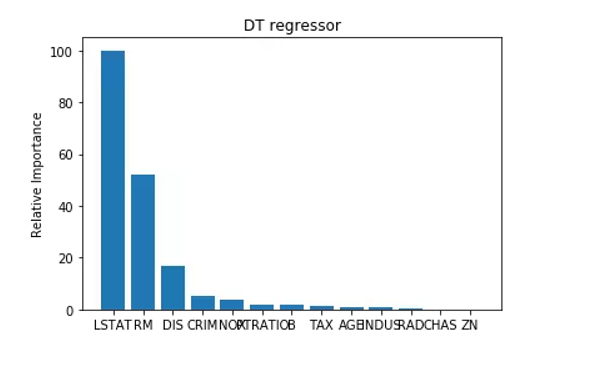## 机器学习中用决策树回归器,构建评估模型 (波士顿房价) 转

o
osc_gu9d45li

1）Graphviz 0.8.4 (安装python模块，安装代码: pip install graphviz)

3）配置环境变量：D:\Program Files (x86)\Graphviz2.38\bin

import sklearn
import numpy as np
from matplotlib import pyplot as plt
import pandas as pd
import matplotlib
%matplotlib inline
matplotlib.rcParams['font.sans-serif']=['SimHei']
#分割数据集要导的包
from sklearn.model_selection import train_test_split

from sklearn import datasets              # sklearn自带的datasets中就有Boston房价数据集.
# print(housing_data)

dataset_X=housing_data.data              # 获取影响房价的特征向量，作为feaure X
dataset_y=housing_data.target             # 获取对应的房价，作为label y
#查看一下他的行与列
print(dataset_X.shape)

#分割数据集,把测试数据集和训练数据集分开
train_X,test_X,train_Y,test_Y = train_test_split(dataset_X,dataset_y,test_size=0.2,random_state=30)    #这样,就把数据(2,8)分成了测试集与训练集

#训练集为80%,测试集为20%
print(train_X.shape,test_X.shape)

# 构建决策树模型
from sklearn.tree import DecisionTreeRegressor
# 决定决策树的最大深度,为 4, 关于这个深度,有兴趣了解的同学,可以到这里看一看  ::::    https://www.cnblogs.com/fionacai/p/5894142.html
decision_regressor =DecisionTreeRegressor(max_depth=4)
#训练数据
decision_regressor.fit(train_X,train_Y)

#使用测试集来判断他的好坏
predict_test_y = decision_regressor.predict(test_X)

#决策树模型得分指标    这是以测试集为标准进行估测
from sklearn import metrics
print('平均绝对误差:{}'.format(metrics.mean_squared_error(predict_test_y,test_Y)))
print('均方误差MSE:{}'.format(metrics.mean_absolute_error(predict_test_y,test_Y)))
print('解释方差分:{}'.format(metrics.explained_variance_score(predict_test_y,test_Y)))
print('R2得分:{}'.format(metrics.r2_score(predict_test_y,test_Y)))

决策树模型的优化
#优化你的模型,让他的得分更高,这样预测出来的数据会更准确
for depth in range(2,12):
decision_regressor_test=DecisionTreeRegressor(max_depth=depth)
decision_regressor_test.fit(train_X,train_Y)
predict_test_y2=decision_regressor_test.predict(test_X)

#优化后的决策树模型得分指标
from sklearn import metrics
print('平均绝对误差:{}'.format(metrics.mean_squared_error(predict_test_y2,test_Y)))
print('均方误差MSE:{}'.format(metrics.mean_absolute_error(predict_test_y2,test_Y)))
print('解释方差分:{}'.format(metrics.explained_variance_score(predict_test_y2,test_Y)))
print('R2得分:{}'.format(metrics.r2_score(predict_test_y2,test_Y)))

计算不同特征的相对重要性

#  计算不同特征的相对重要性
def plot_importances(feature_importances, title, feature_names):
''将feature_importance绘制到图表中，便于观察,并把重要性大于5的特征打印出来'''
# 将重要性都归一化为0-100之内
feature_importances=100.0*(feature_importances/max(feature_importances))
#将得分从高到低排序
# (np.argsort:将矩阵a按照axis排序(默认升序)，并返回排序后的下标 )
# (np.flipud:矩阵上下翻转，np.fliplr:矩阵左右反转)
index_sorted=np.flipud(np.argsort(feature_importances))
# 让X坐标轴上的标签居中显示
pos=np.arange(index_sorted.shape)+0.5
# 画条形图
plt.figure()
plt.bar(pos,feature_importances[index_sorted],align='center')
plt.xticks(pos,feature_names[index_sorted])
plt.ylabel('Relative Importance')
plt.title(title)

# 把重要性结果打印出来
print('{} importance list------>>>>>'.format(title))
for importance,name in zip(feature_importances[index_sorted],feature_names[index_sorted]):
if  importance>5:
print('feature:{}, importance: {:.2f}'.format(name,importance))
decision_regressor7=DecisionTreeRegressor(max_depth=7)      # 最大深度确定为7
decision_regressor7.fit(train_X,train_y)       # 对决策树回归模型进行训练
plot_importances(decision_regressor7.feature_importances_,
'DT regressor',housing_data.feature_names)o

### osc_gu9d45li#### 暂无文章

Java线程池

nullpointerxyz
17分钟前
29
0
Python笔记：用Python制作二维码

tengyulong
29分钟前
0
0
Redis-初体验/数据结构

31分钟前
15
0

fyin1314

29
0

19
0